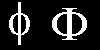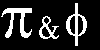Search This Site The Web forHome
New to LucyTuning?
Academic & Research, John "Longitude" Harrison
Products & Services
LucyTuned Lullabies
Guitars & Frets
Midi, Keyboards & Composition
Colour & Mapping
Comparison to other Tuning Systems
Music Theory
Press Room
Listening Room
 Pi is the ratio of the diameter to the circumference of a circle. It is usually represented by the Greek letter pi. There are many ways to derive the value and the calculation of pi has been refined during history from a simple 22/7 ratio to computer calculations running to many millions of digits. Pi is both irrational, (its infinitely long string of decimal places never repeats), and transcendental, (it is not a solution of any polynomial equation with whole number coefficients), occurs naturally and is used in all known cultures.3.14159 26535 89793 23846 26433 83279 50288 41971 etc.. There are many ways to calculate this unique number. For example: * By continuing the series 1 - 1/3 + 1/5 - 1/7 + 1/9 and multiplying the result by four gives pi. * Adding the reciprocals of the squares (i.e. 1/1 + 1/4 + 1/9 + 1/16 + 1/25 ....) gives (pi^2)/6.Phi is also known as the Golden or Divine Ratio, and has been used in many models for patterns found in nature and artistic concepts. Mathematically, it is half of the square root of five, plus one.... (5^(1/2)+1)/2 = 1.618034..... Phi may be derived from the Fibonacci series, as it is the ratio of increase from the series: 1,2,3,5,8,13,21,34,55,89,144............ Phi is the only number which is one greater that its reciprocal. phi = 1 + (1/phi) which is 1.618...... = 1 + (1/1.618.....)Pi and Phi are connected mathematically, for pi may be calculated from the Fibonacci sequence The Fibonacci sequence is defined by the formula u(0) = 0, u(1) = 1 u(n+1) = u(n) + u(n-1) So u(2) = 1, u(3) = 2, u(4) = 5, u(5) = 8, u(6) = 13, u(7) = 21, u(8) = 34......... This connects to pi in the following manner: Calculate all the Fibonacci numbers from u(1) up to u(m). Multiply all the results together and call this P(m). Find the Least Common Multiple LCM(m) from the numbers u(1) through u(m). (The Least Common Multiple of two or more numbers is the smallest number that can be exactly divided by all the numbers) Calculate the square root of 6logP(m)/logLCM(m) and call it Z(m). As values of m increases the value of Z(m) becomes closer to pi. For m=7 P(m) is 1*1*2*3*5*8*13*21 = 32760 LCM(m)=10920 (i.e. 3*5*7*2*2*2*13) 6*(log32760/log10920) = 6*4.515/4.038 = 6.709. Square root is 2.590 = Z(m) For m=8 P(m) is 1*1*2*3*5*8*13*21*34= 1113840 LCM(m)=185640 6*(log1113840/log185640) = 6*6.0468/5.2687= 6.886. Square root is 2.6241 = Z(m) This serves to show the method for low numbers. As the values of m increase eventually Z(m) reaches pi. A sort-of-related afterthought: A formula found by the Swiss mathematician Leonhard Euler in 1748, states that when one is added to the transcendental number "e" (roughly 2.71828) raised to the power of Pi (roughly 3.14159) multiplied by the square-root of minus one, the result is precisely zero.